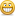# A Solution to rounding in Storyline – without using Javascript

Normal 0 false false falseoNotPromoteQF /> EN-US X-NONE X-NONE

I found a way to round numbers in Storyline without using Javascript.  The challenge was to show whole percentage numbers from calculated decimal numbers (i.e. 33% for 1/3 and 67% for 2/3).  Searching through the forums, all I could find was Javascript solutions and suggestions to submit a feature request.  Not happy with either of these answers, I continued to play around.

I found that Storyline only holds 7 digits past the decimal place, and the 7th digit is rounded.  So to get a rounded decimal (percentage) as a whole number, divide by 100,000, and then multiply by 10,000,000.

For example:

2 / 3 = 0.6666667  (rounded at the 7th position by Storyline)

0.6666667 / 100,000 = .000006666667 (which is rounded to 0.0000067)

0.0000067 * 10,000,000 = 67

Now we have our rounded 67% from 2/3

You should be able to apply this to any number you want to round.  Divide and then multiply by a multiple of 10 that is large enough to push the number you want to round to the 7th position past the decimal (i.e. 13,567 / 10,000,000,000 * 10,000,000,000 = 14,000)

###### 95 RepliesTotally confused. I got this working yesterday on one course (screenshot where the results slide says 4.3). Did the same thing on another course (slide 1.45) and can't get it to work! It all looks the same to me but I keep getting two decimals. On a side note, has anyone else had issues when copying a result slide from one course to another? Seems to mess up the variables. I had to rebuild the second course's result screen from scratch (I could paste the objects, layers and triggers though). Help!Quite genius actually.  It does add or subtract one, but does it while the condition is true.  So in the add case, it adds one until the condition "rounded < original_score" is not true anymore.  So in the case of 13.76, it keeps adding a whole number 14 times, to give you 14.  If you change the "original_score" variable to any decimal number, you can see that value change.